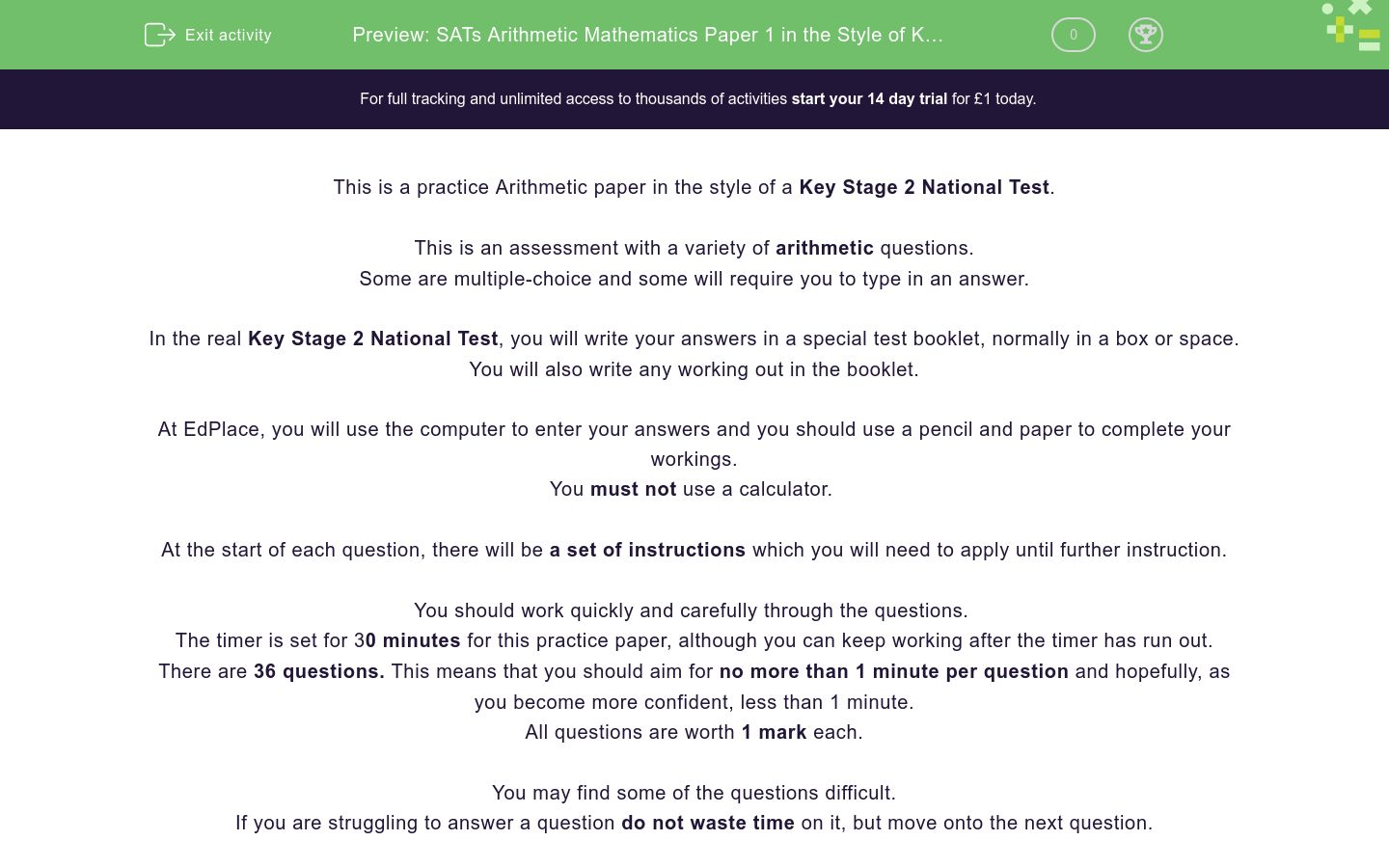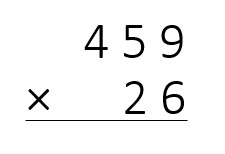# SATs Arithmetic Mathematics Paper 1 in the Style of Key Stage 2 National Tests (Practice 1)

In this assessment, students will be able to complete a timed Arithmetic paper in the style of Key Stage 2 SATs.Key stage:  KS 2

Curriculum topic:   SATs Practice Papers

Curriculum subtopic:   Arithmetic Practice Papers

Difficulty level:### QUESTION 1 of 10

This is a practice Arithmetic paper in the style of a Key Stage 2 National Test.

This is an assessment with a variety of arithmetic questions.

Some are multiple-choice and some will require you to type in an answer.

In the real Key Stage 2 National Test, you will write your answers in a special test booklet, normally in a box or space.

You will also write any working out in the booklet.

At EdPlace, you will use the computer to enter your answers and you should use a pencil and paper to complete your workings.

You must not use a calculator.

At the start of each question, there will be a set of instructions which you will need to apply until further instruction.

You should work quickly and carefully through the questions.

The timer is set for 30 minutes for this practice paper, although you can keep working after the timer has run out.

There are 36 questions. This means that you should aim for no more than 1 minute per question and hopefully, as you become more confident, less than 1 minute.

All questions are worth 1 mark each.

You may find some of the questions difficult.

If you are struggling to answer a question do not waste time on it, but move onto the next question.

Disclaimer:

We have no affiliation to the Standards and Testing Agency (STA) and these questions represent our own unique content developed by EdPlace Key Stage 2 Maths authors.

None of the content displayed here has been supplied by the STA or any other third party suppliers.

? = 8000 + 65

956 = 900 + ? + 6

? = 5256 + 56

5.26 + 2.156 = ?

81 × 11 = ?

? + 7 = 232

516 × 0 = ?

240 ÷ 30 = ?

84 ÷ 6 = ?

? = 97 - 15

640 ÷ 64 = ?

1000 - ? = 899

1440 ÷ 12 = ?

3.596 × 100 = ?

9² = ?

Find 20% of 600.

64 ÷ (2 × 4) = ?

216 ÷ 1000 = ?

4 - 2.55 = ?

6 - 2.9 = ?

1.3 × 100 = ?Select the correct whole number and fraction to show the answer as a mixed number.

Solve this long multiplication calculation:Type the answer in the boxes showing any remainders as r.Choose a whole number and fraction from the options to give the correct answer as a mixed number in its simplest form.

22% of £90 = ?

Type the answer in the box below showing any pence after the decimal point.

Make sure your answer has 2 decimal places as it is showing an amount of money.Type the answer in the boxes below showing the answer as a fraction in its simplest form.

33% of 700 = ?Choose from the options below to show your answer as a mixed number in its simplest form.23% of 450 = ?Type your answer in the boxes below, keeping the numerator and denominator separate.60% × 540 = ?

• Question 1

? = 8000 + 65

EDDIE SAYS
The answer is 8065. Zero holds the place in the hundreds column.
• Question 2

956 = 900 + ? + 6

EDDIE SAYS
The missing number is 50 because 900 + 50 + 6 = 956.
• Question 3

? = 5256 + 56

EDDIE SAYS
• Question 4

5.26 + 2.156 = ?

EDDIE SAYS
• Question 5

81 × 11 = ?

EDDIE SAYS
The missing number is 891. 81 × 10 = 810 810 + 81 - 891
• Question 6

? + 7 = 232

EDDIE SAYS
The answer is 825. Use the inverse to find the missing number. 232 - 7 = 225
• Question 7

516 × 0 = ?

EDDIE SAYS
The answer is 0. Whenever a number is multiplied by zero, the answer will also be zero.
• Question 8

240 ÷ 30 = ?

EDDIE SAYS
The answer is 8. 3 × 8 = 24 So, 30 × 8 = 240
• Question 9

84 ÷ 6 = ?

EDDIE SAYS
• Question 10

? = 97 - 15

EDDIE SAYS
• Question 11

640 ÷ 64 = ?

EDDIE SAYS
The missing number is 10 because 64 fits into 640 ten times.
• Question 12

1000 - ? = 899

EDDIE SAYS
The missing number is 101.
• Question 13

1440 ÷ 12 = ?

EDDIE SAYS
The answer is 120. 114 ÷ 12 = 12 So, 1140 ÷ by 12 = 120
• Question 14

3.596 × 100 = ?

EDDIE SAYS
The missing number is 359.6. When multiplying by 100, all the digits move to the left 2 places.
• Question 15

9² = ?

EDDIE SAYS
The missing number is 81. 9² means 9 × 9 (or nine squared).
• Question 16

Find 20% of 600.

EDDIE SAYS
20% of 600 is 120. First, find 10% by dividing by 10. 600 ÷ 10 = 60 Next, double 60 to find 20%. 60 × 2 = 120
• Question 17

64 ÷ (2 × 4) = ?

EDDIE SAYS
The missing number is 8. Always work out the brackets first. 64 ÷ (2 × 4) 2 × 4 = 8 64 ÷ 8 = 8
• Question 18

216 ÷ 1000 = ?

EDDIE SAYS
The answer is 0.216. When dividing by 1000, all digits move 3 places to the right.
• Question 19

4 - 2.55 = ?

EDDIE SAYS
The missing number is 1.45.
• Question 20

6 - 2.9 = ?

EDDIE SAYS
The missing number is 3.1.
• Question 21

1.3 × 100 = ?

EDDIE SAYS
The missing number is 130. When multiplying by 100, each digit moves 2 places to the left.
• Question 22Select the correct whole number and fraction to show the answer as a mixed number.

EDDIE SAYS
The missing fraction is a mixed number of 1 4/7 2 2/7 is the same as 16/7. 16/7 - 5/7 = 11/7 - 1 4/7
• Question 23

Solve this long multiplication calculation:11934
EDDIE SAYS
• Question 24EDDIE SAYS
The missing fraction is 5/6. Use the common multiple of 6 to make both the fractions have the same denominator. 2/3 becomes 4/6. 1/6 + 4/6 = 5/6
• Question 25Type the answer in the boxes showing any remainders as r.

EDDIE SAYS
The missing number is 132 r9.
• Question 26Choose a whole number and fraction from the options to give the correct answer as a mixed number in its simplest form.

EDDIE SAYS
The answer in its simplest form is 4 1/4. First, add the whole numbers. 1 + 3 = 4 Next, convert the fractions so they both have the same denominator. 1/5 = 4/20. Then, add the fractions. 4/20 + 1/20 = 5/20 So, altogether the answer is 4 5/20, which simplifies to 4 1/4.
• Question 27

22% of £90 = ?

Type the answer in the box below showing any pence after the decimal point.

Make sure your answer has 2 decimal places as it is showing an amount of money.

EDDIE SAYS
The answer is £19.80. 10% of £90 would be £9. 1% of £90 would be 90p. 20% would be £18. 2% would be £1.80. £18 + £1.80 = £19.80.
• Question 28Type the answer in the boxes below showing the answer as a fraction in its simplest form.

EDDIE SAYS
The answer is 9/40. First, convert the fractions using the lowest common multiples of 40. 5/8 becomes 25/40. 2/5 becomes 16/40. 25/40 - 16/40 = 9/40 which cannot be simplified.
• Question 29

33% of 700 = ?

231
EDDIE SAYS
The answer is 231. 10% of 700 = 70. 1% of 700 = 7 30% = 210 3% - 21 210 + 21 = 231
• Question 3056,134
56134
EDDIE SAYS
• Question 31Choose from the options below to show your answer as a mixed number in its simplest form.

EDDIE SAYS
The answer is 1 3/5. 4 is the same as 4/1. So, multiply the numerators first: 2 × 4 = 8 5 × 1 = 5 The answer is 8/5 or 1 3/5 as a mixed number. It cannot be simplified.
• Question 32EDDIE SAYS
The answer is 198 r 14. 23 × 198 = 4554 4568 - 4554 = 14
• Question 33

23% of 450 = ?

103.5
EDDIE SAYS
The answer is 103.5. 10% of 450 = 45. 1% of 450 = 4.5. 20% = 90 3% = 13.5 90 + 13.5 = 103.5
• Question 34Type your answer in the boxes below, keeping the numerator and denominator separate.

EDDIE SAYS
The answer is 2/21. 2/7 ÷ 3 is the same as 2/7 ÷ 3/1. To divide a fraction by a fraction, change the divide symbol to a multiply symbol and flip the second fraction. 2/7 × 1/3 = 2/21
• Question 3568
EDDIE SAYS
The answer is 68. This calculation means the same as finding 4/6 of 102. To find 1/6 divide the whole number by 6. 1/6 is 17. 17 × 4 = 68
• Question 36

60% × 540 = ?

324
EDDIE SAYS
The answer is 324. 60% × 540 means the same as finding 60% of 540. 10% of 540 is 54. 6 × 54 = 324.
---- OR ----

Sign up for a £1 trial so you can track and measure your child's progress on this activity.

### What is EdPlace?

We're your National Curriculum aligned online education content provider helping each child succeed in English, maths and science from year 1 to GCSE. With an EdPlace account you’ll be able to track and measure progress, helping each child achieve their best. We build confidence and attainment by personalising each child’s learning at a level that suits them.

Get started